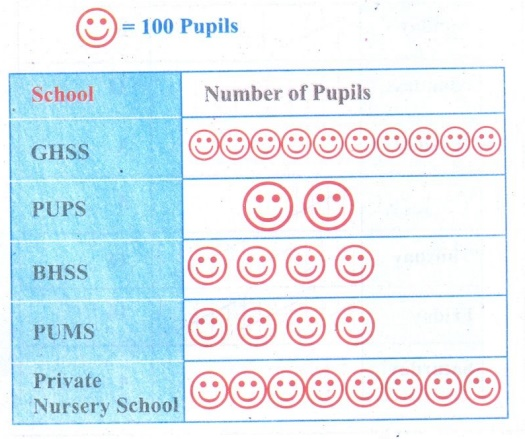Home | | Maths 5th Std | Exercise 6.2 (Pictograph)

# Exercise 6.2 (Pictograph)

Text Book Back Exercises Questions with Answers, Solution : 5th Maths : Term 1 Unit 6 : Information Processing : Exercise 6.2 (Pictograph)

Exercise 6.2

1. The following table shows the weight of paddy Cultivated in a particular village between 2010 and 2015Observe the pictograph and answer the following questions.

1. In which year the paddy production was maximum? 2010

2. In which years the paddy productions were equal?

2012 and 2014,

2013 and 2015

3. Find the paddy production in 2015. 200 kg

4. Find the total quantity of paddy production in 2013, 2014, and 2015. 700kg

2. The total number of pupils studying in class 5 are as follows

GHSS: 1000

PUPS: 200

BHSS: 400

PUMS: 400

Private nursery School : 800

Prepare a pictograph using the symbolto represent 100 Pupils and answer the following question:1. Which school has the maximum number of pupils?

1. GHSS has the maximum number of pupils.

2. Which school has the least number of pupils?

2. PUPS has the least number of pupils.

Tags : Information Processing | Term 1 Chapter 6 | 5th Maths , 5th Maths : Term 1 Unit 6 : Information Processing
Study Material, Lecturing Notes, Assignment, Reference, Wiki description explanation, brief detail
5th Maths : Term 1 Unit 6 : Information Processing : Exercise 6.2 (Pictograph) | Information Processing | Term 1 Chapter 6 | 5th Maths